Free Printable Worksheets for Second Grade

Introduction to Programming: Plot the Robot’s Path – 2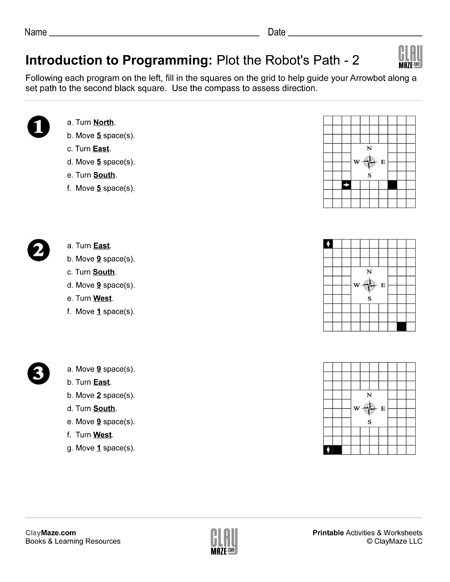Learning to interact with computers and equipment at an early edge is critical for today’s children. They are expected to interface and adapt to computers at an earlier and earlier age and any chan...

Word Problems I: Addition & Subtraction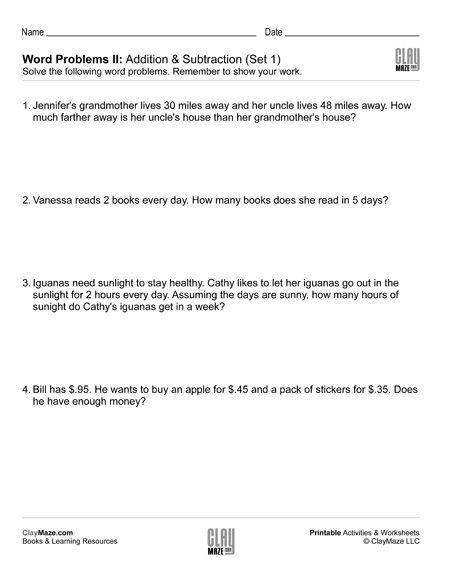These are sets of basic addition and subtraction word problems. The student should read through each problem thoroughly to answer the questions and be sure to show their work below each one. These ...

Word Problems II: Addition & SubtractionThese sets of word problems are slightly more challenging than the Word Problems I sets. The student will need to use addition and subtraction to solve, but some problems use a little bit larger nu...

Time Word Problems: Analog ClocksThese sets of worksheets are great for practice telling time with analog clocks and for understanding how to add and subtract with different time intervals. On these worksheets, the student is aske...

Subtraction Worksheet – 3 Digit Subtraction with Regrouping (Set A)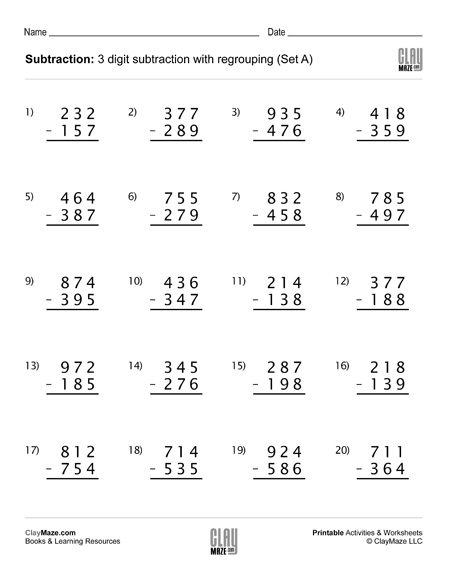This worksheet contains 20 subtraction problems with regrouping. There is extra spacing between the digits for borrowing....

Subtraction Worksheet – 3 Digit Subtraction with Regrouping (Set B)Download this worksheet for subtraction practice with regrouping. The problems use 3 digit numbers with spacing between the digits to allow room for borrowing. There are 20 problems....

Subtraction Worksheet – 3 Digit Subtraction with Regrouping (Set C)Use this 3 digit subtraction worksheet with regrouping for lots of practice. There are 20 problems and numbers have enough spacing between the digits for borrowing....

Subtraction Worksheet – 3 Digit Subtraction with Regrouping (Set D)3 digit subtraction worksheet with regrouping – 20 problems. There is extra spacing between the digits for borrowing....

Alphabetical Order with Word Bank – Topic: BiologyDownload and print this free worksheet for practice with sorting words in alphabetical order. The topic is biology and the words in the word bank are biology terms....

Alphabetical Order with Word Bank – Topic: Chemistry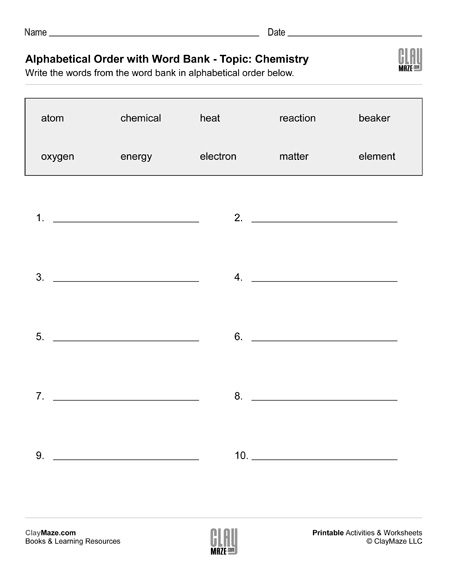In this worksheet the student is asked to alphabetize the words in the word bank. The topic of this worksheet is chemistry....

Alphabetical Order with Word Bank – Topic: GeographyWrite the words from the word bank in alphabetical order. The topic of the words in this worksheet is geography....

Alphabetical Order with Word Bank – Topic: Insects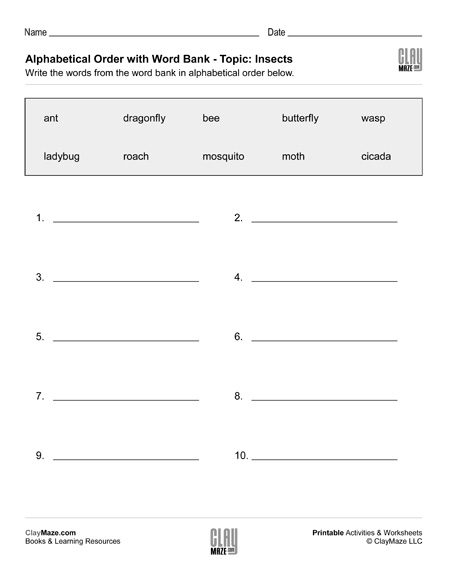Write the words from the word bank in alphabetical order. The words in this worksheet are all about insects....

Even Numbers and Odd Numbers (Set 1)This worksheet is for practice identifying even numbers and odd numbers. It is divided into 2 sections. The student is asked to circle the even numbers in each group in the first section and then...

Even Numbers and Odd Numbers (Set 2)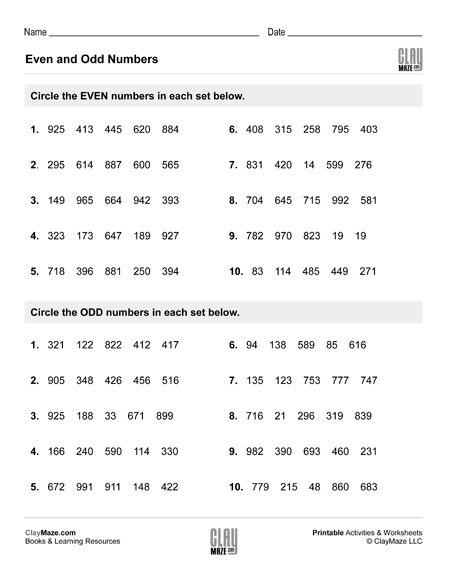Even and odd numbers worksheet. In the first section, the student is asked to circle the even numbers in each group. Then in the second section the student is asked to circle the odd numbers in...

Even Numbers and Odd Numbers (Set 3)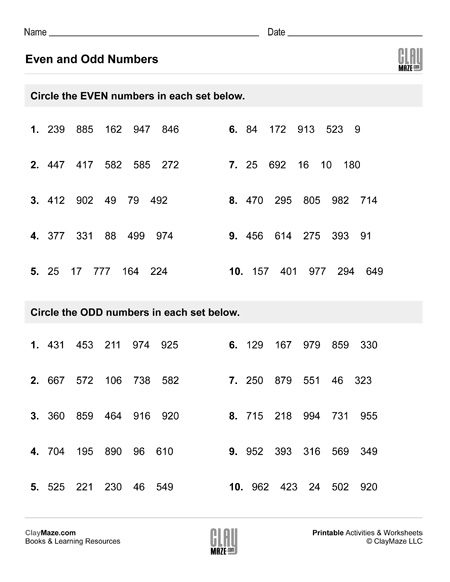Use this worksheet for practice with even numbers and odd numbers. Find the even numbers in each set of the first section, then find the odd numbers in each set of the second section....